# How to use adjustable and big curly bracket in LaTeX?

In LaTeX, you will always use curly brackets. But, you cannot use direct like other brackets while using in document.

\documentclass{article}
\begin{document}
${a_1,a_2, \cdots, a_n}$
$(b_1,b_2, \cdots, b_n)$
$[c_1,c_2, \cdots, c_n]$
$|d_1,d_2, \cdots, d_n|$
\end{document}

Output :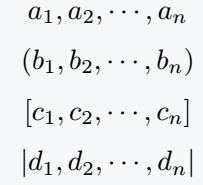Solution to this problem is to use a backslash before both curly brackets. Let’s see if it is executed or not!

\documentclass{article}
\usepackage{amssymb}
\begin{document}
${a_1}, \{a_2\}, {a_3}, \{a_4\}$
$S_n = \{1,2,3,\cdots, n\}$
$S_n = \{a_1,a_2,a_3,\cdots, a_n\}$
% Don't used adjustable curly bracket
$\mathbb{Q} = \{ \frac{p}{q} | a,b \in \mathbb{Z}, b\neq 0 \}$
$\{\frac{a_1}{n}\} , \{\frac{b_1}{m}\} , \{\frac{c_1}{k}\}$
\end{document}

Output :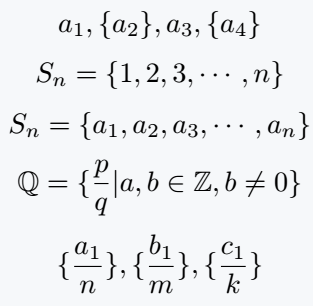## Adjustable size of curly bracket

In the code above, a mathematical expression was given to you to understand, where size of mathematical equation was larger than curly brackets.

It seems like a big problem to you but its solution is very simple. For this, LaTeX has a built-in command that adjusts size of bracket according to size of expression.

\documentclass{article}
\begin{document}
$\{a_1\}, \left\{\frac{a_2}{k}\right\}, \{a_3\}, \left\{\frac{a_4}{k}\right\}$
$S_n = \left\{\frac{n(n+1)}{2}\right\}$
$S_n =\left\{\cfrac{\sum\limits_{i=1}^{n} {s_i}}{n}\right\}$
$\mathbb{Q} = \left\{ \frac{p}{q} | a,b \in \mathbb{Z}, b\neq 0 \right\}$
$\left\{\frac{a_1}{n_1}\right\} , \left\{\frac{b_1}{m_1}\right\} , \left\{\frac{c_1}{k_1}\right\}$
\end{document}

Output :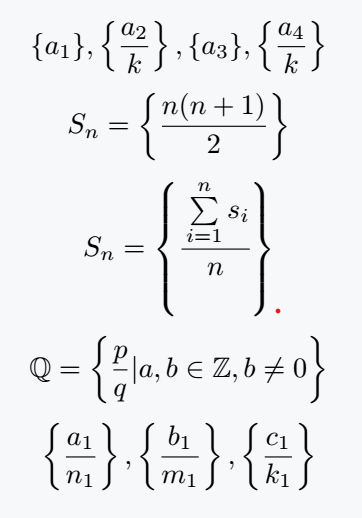Second, you can manually increase size of bracket, but there are limitations in this case.

\documentclass{article}
\begin{document}
$\big\{\sigma\big\} \; \Big\{\sigma_{ij}\Big\} \; \bigg\{\frac{\sigma}{n}\bigg\} \Bigg\{\frac{\sigma_{ij}}{n_k}\Bigg\}$
$\big\{ \Big\{ \bigg\{ \Bigg\{ x \Bigg\} \bigg\} \Big\} \big\}$
$\big\{ r \big\} \Big\{ e \Big\} \bigg\{ a \bigg\} \Bigg\{ d\Bigg\}$
\end{document}

Output :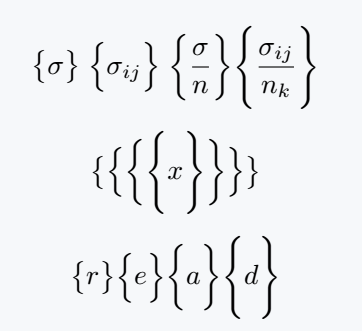## Use newcommand

If you frequently use a specific size of curly bracket in your documents, you can define a new command using the “\newcommand” command. This allows you to define a custom command that can be easily reused throughout your document.

\documentclass{article}
\newcommand{\curly}{\left\{ #1 \right\}}
\newcommand{\curlb}{#1\{ #2 #1\}}
\begin{document}
$\curly{x} \curly{\frac{x}{y}}$
$\curly{\frac{n(n+1)}{n}} \curly{\frac{a}{b}}$
$\curlb{\big}{a} \curlb{\Big}{b} \curlb{\bigg}{c} \curlb{\Bigg}{d}$
\end{document}

Output :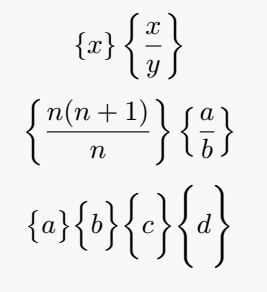## Use physics package

Many of you may be familiar with the physics package. But, did you know that this package has an in-built command for curly brackets! Let’s see its use

\documentclass{article}
\usepackage{physics}
\begin{document}
$\qty{x} \; \qty{\frac{x}{y}}$
$\qty{\frac{n^2 + 1}{n}}$
$\qty\big{m} \qty\Big{a} \qty\bigg{t} \qty\Bigg{h}$
$\Bqty{\frac{n^2 + 2n + 1}{n}}$
\end{document}

Output :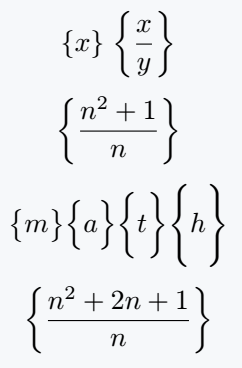For \qty command, curly brackets will be automatically adjustable with expressions.

Along with \qty command, you can use the big command. But, in this case the process of using big command is completely different.

## Use curly bracket in Matrix

You’ll notice that matrices are bounded by curly brackets. You don’t need to use separate brackets for this. Just pass Bmatrix argument to matrix environment.

\documentclass{article}
\usepackage{amsmath}
\begin{document}
$\begin{Bmatrix} a_{11} & a_{12} & \cdots & a_{1n}\\ a_{21} & a_{22} & \cdots & a_{2n}\\ \vdots & \vdots & \ddots & \vdots\\ a_{m1} & a_{m2} & \cdots & a_{mn} \end{Bmatrix} \times \begin{Bmatrix} b_{11} & b_{12} & \cdots & b_{1p}\\ b_{21} & b_{22} & \cdots & b_{2p}\\ \vdots & \vdots & \ddots & \vdots\\ b_{n1} & b_{n2} & \cdots & b_{np} \end{Bmatrix} = \begin{Bmatrix} c_{11} & c_{12} & \cdots & c_{1p}\\ c_{21} & c_{22} & \cdots & c_{2p}\\ \vdots & \vdots & \ddots & \vdots\\ c_{m1} & c_{m2} & \cdots & c_{mp} \end{Bmatrix}$
\end{document}

Output :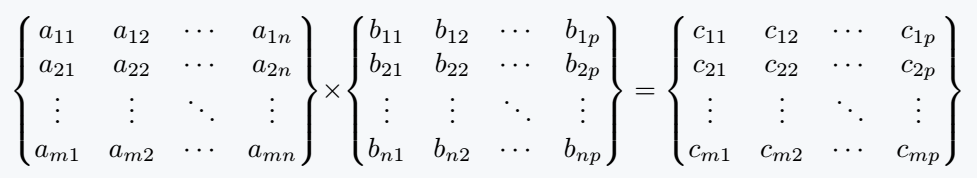#### Md Jidan Mondal

LaTeX expert with over 10 years of experience in document preparation and typesetting. Specializes in creating professional documents, reports, and presentations using LaTeX.